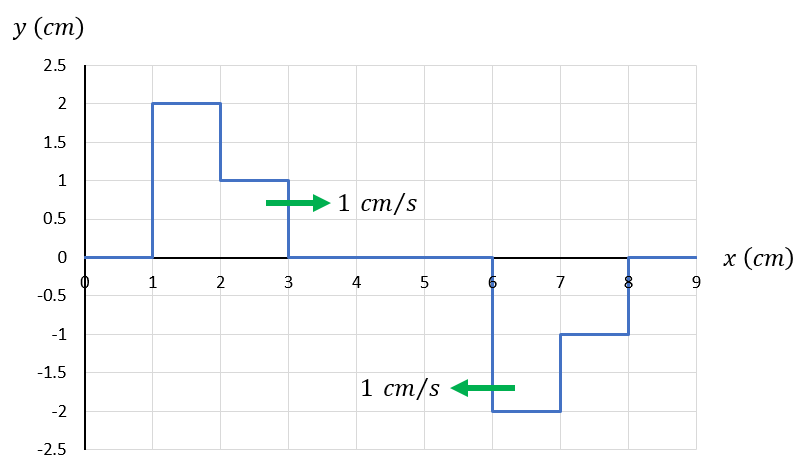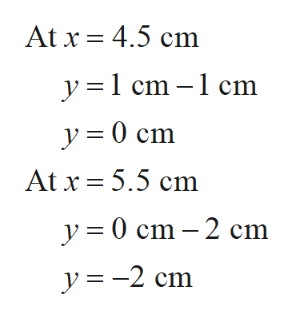# у (ст)2.521.511 ст/s0.5х (ст)0123457-0.5-1-1.51 ст/s-2-2.5— о-

Question
17 views

Two wave pulses travel on a string. The picture shows the displacement of the string y as a function of x at t = 0. The velocities of the wave pulses are shown.

1. At t = 2 s, what is the displacement of the string at the following values of x?

a. At x = 4.5 cm, y =  _____ cm
b. At x = 5.5 cm, y =  _____ cm

2. At t = 3 s, what is the displacement of the string at the following values of x?

a. At x = 3.5 cm, y = ______  cmhelp_outlineImage Transcriptioncloseу (ст) 2.5 2 1.5 1 1 ст/s 0.5 х (ст) 0 1 2 3 4 5 7 -0.5 -1 -1.5 1 ст/s -2 -2.5 — о- fullscreen
check_circle

Step 1

Consider the vertical axis denotes displacement(y) of the wave as the function of x (horizontal axis).

1. consider the situation at t=2 s.
Step 2

The total displacement can be calculate by taking the algebraic sum of the both wave at that instant(x).help_outlineImage TranscriptioncloseAt x 4.5 cm y =1 cm -1 cm y0 cm At x 5.5 cm y 0 cm 2 cm y-2 cm fullscreen
Step 3
1. consider the situation at t=3 s....

### Want to see the full answer?

See Solution

#### Want to see this answer and more?

Solutions are written by subject experts who are available 24/7. Questions are typically answered within 1 hour.*

See Solution
*Response times may vary by subject and question.
Tagged in

### Other